# Calculator fractions multiple

The calculator performs basic and advanced operations with fractions, expressions with fractions combined with integers, decimals, and mixed numbers. It also shows detailed step-by-step information about the fraction calculation procedure. Solve problems with two, three, or more fractions and numbers in one expression.

### 2/3 * 3/5 = 2/5 = 0.4

Spelled result in words is two fifths.

### How do you solve fractions step by step?

1. Multiple: 2/3 * 3/5 = 2 · 3/3 · 5 = 6/15 = 2 · 3/5 · 3 = 2/5
Multiply both numerators and denominators. Result fraction keep to lowest possible denominator GCD(6, 15) = 3. In the next intermediate step, , cancel by a common factor of 3 gives 2/5.
In words - two thirds multiplied by three fifths = two fifths.

#### Rules for expressions with fractions:

Fractions - use the slash “/” between the numerator and denominator, i.e., for five-hundredths, enter 5/100. If you are using mixed numbers, be sure to leave a single space between the whole and fraction part.
The slash separates the numerator (number above a fraction line) and denominator (number below).

Mixed numerals (mixed fractions or mixed numbers) write as non-zero integer separated by one space and fraction i.e., 1 2/3 (having the same sign). An example of a negative mixed fraction: -5 1/2.
Because slash is both signs for fraction line and division, we recommended use colon (:) as the operator of division fractions i.e., 1/2 : 3.

Decimals (decimal numbers) enter with a decimal point . and they are automatically converted to fractions - i.e. 1.45.

The colon : and slash / is the symbol of division. Can be used to divide mixed numbers 1 2/3 : 4 3/8 or can be used for write complex fractions i.e. 1/2 : 1/3.
An asterisk * or × is the symbol for multiplication.
Plus + is addition, minus sign - is subtraction and ()[] is mathematical parentheses.
The exponentiation/power symbol is ^ - for example: (7/8-4/5)^2 = (7/8-4/5)2

The calculator follows well-known rules for order of operations. The most common mnemonics for remembering this order of operations are:
PEMDAS - Parentheses, Exponents, Multiplication, Division, Addition, Subtraction.
BEDMAS - Brackets, Exponents, Division, Multiplication, Addition, Subtraction
BODMAS - Brackets, Of or Order, Division, Multiplication, Addition, Subtraction.
GEMDAS - Grouping Symbols - brackets (){}, Exponents, Multiplication, Division, Addition, Subtraction.
Be careful, always do multiplication and division before addition and subtraction. Some operators (+ and -) and (* and /) has the same priority and then must evaluate from left to right.

## Fractions in word problems:

• The tap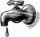For one day flows 148 l of water out of the tap. How much water will flow out for 3/4 day?
• ExpressionsLet k represent an unknown number, express the following expressions: 1. The sum of the number n and two 2. The quotient of the numbers n and nine 3. Twice the number n 4. The difference between nine and the number n 5. Nine less than the number n
• Dice and coinA number cube is rolled and a coin is tossed. The number cube and the coin are fair. What is the probability that the number rolled is greater than 2 and the coin toss is head?
• Sewing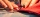Beth's mother can sew 235 pairs of short pants in 6 days while Lourdes can sew 187 pairs in 8 days. How many more pairs of short pants can Beth's mother sew?
• Alcohol risk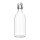The 2009 Youth Risk Behavior Survey found that about 5 out of 12 high school students drank some alcohol in the 30 days preceding the survey. If a high school has 2400 students, how many would be expected to have used alcohol within a 30-day period?
• RickyRicky painted 3/5 of the side of the garage. When he repainted ½ of this part, what part of the side of the garage did he painted twice?
• The kennel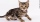20 dogs. Ratio dogs to cats is 3:2  how many cats?
• Golf ballsOf the 28 golf balls, 1/7 are yellow. How many golf balls are yellow? Use the model to help you. Enter your answer in the box.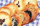In a cookie jar, 1/4 of the cookies are chocolate chip and 1/2 of the rest are peanut butter. What fraction of all the cookies are peanut butter?Issac eats 1/6 of the pizza. Maya then eats 3/5 of the remaining pizza. What fraction of the original pizza is left?Lin has a cake pan that has a length of 8/3 inches, a width of 15/4 inches and a height of 3/2 inches. What is the volume of the cake pan?Max is 1 1/2 times as tall as Sharon. Alan is 4 1/3 feet tall. If Alan is 6 inches taller than Sharon, how tall is Max?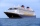Captain Emily has a ship, the H. M. S Crimson Lynx. The ship is five furlongs from the dread pirate Umaima and her merciless band of thieves. If her ship hasn't already been hit, Captain Emily has probability 3/5 of hitting the pirate ship. If her ship ha